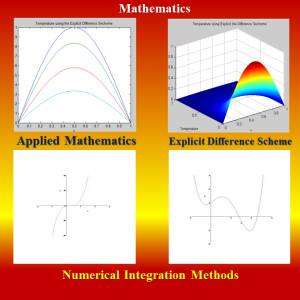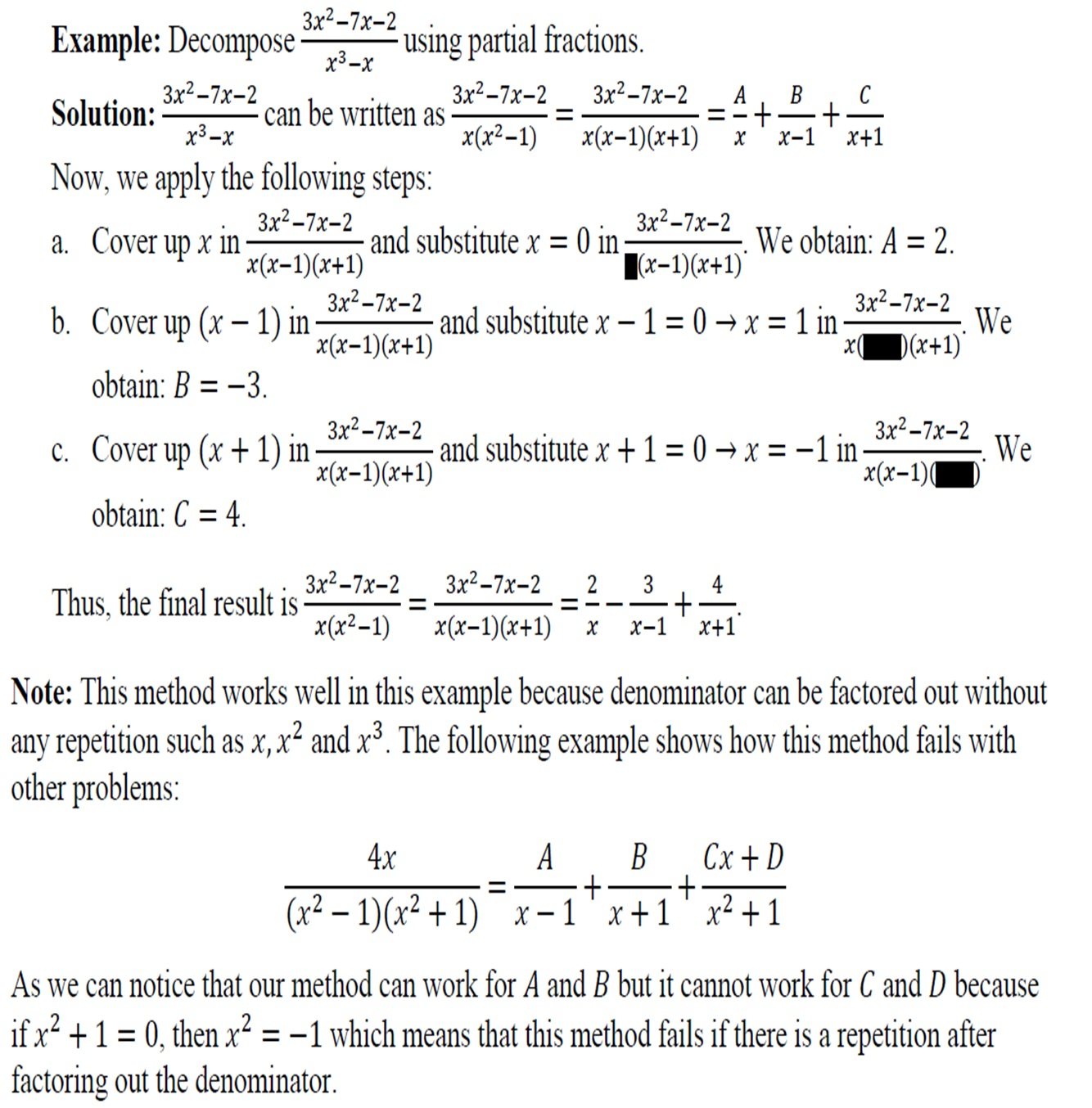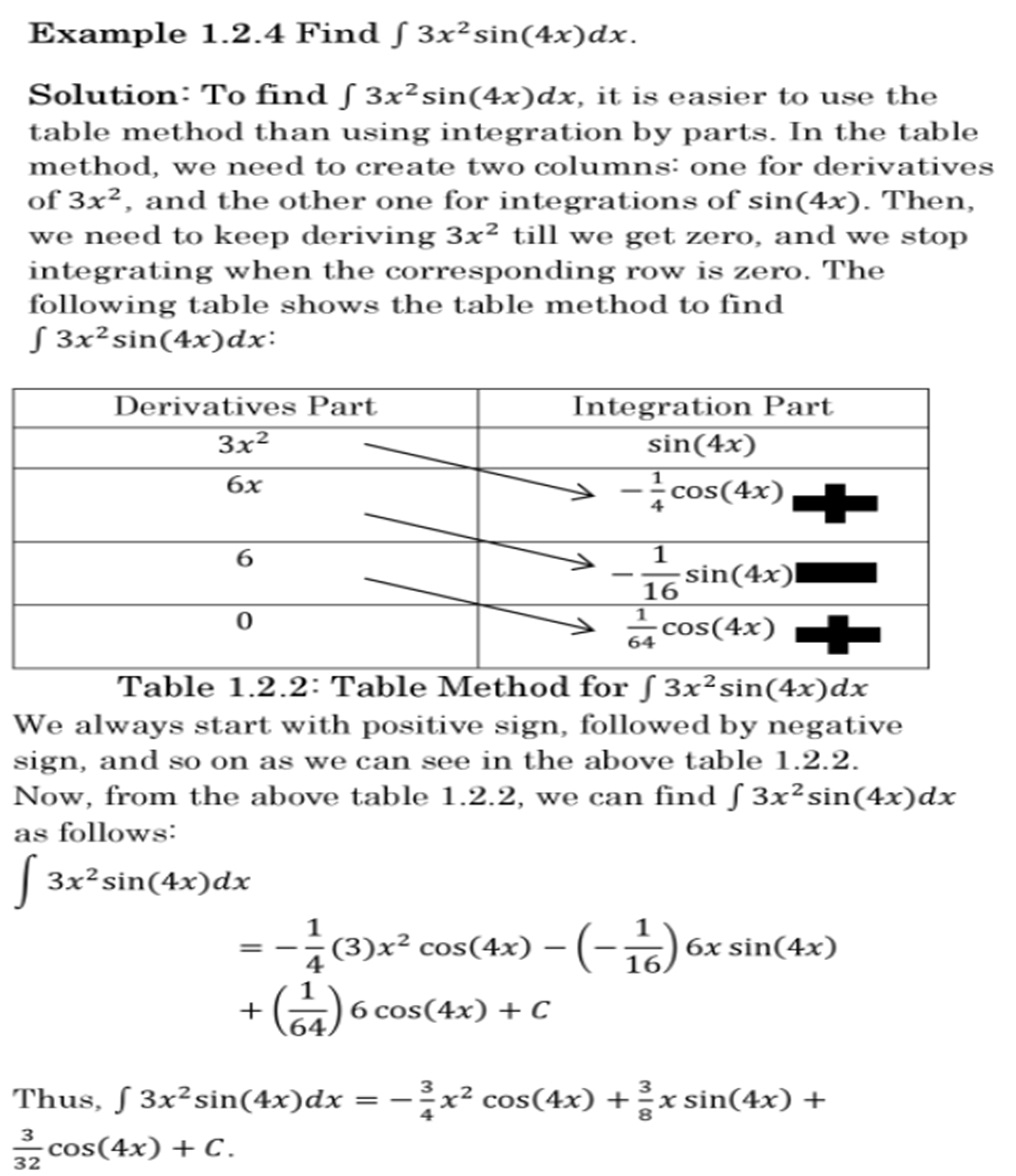# Teaching Calculus: Two Handy Techniques

In my five years of teaching calculus, I’ve noticed that students often struggle with partial fractions and integration by parts. Therefore, here are two alternative methods I use that are faster and easier than the traditional methods that most authors of calculus textbooks use and work on most types of problems:

• The Cover Method: Many of my students struggle with partial fractions because of the complexity of the equations they have to solve. I decided to help them by introducing a new method called the cover method, which can solve about half of the partial fraction decomposition problems:• The Table Method: Integration by parts is considered one of the difficult topics for students of Calculus II because some students either do not memorize the standard form of integration by parts or they do not know how to derive it. To make it easy for my students, I decided to introduce a method called the table method. This method does not require memorization, and it can solve integration by parts problems faster than the traditional method. However, it only works for some integration by parts problems such as ones involving polynomials, exponential functions, and trigonometric functions. The example below is from my textbook A Friendly Introduction to Differential EquationsTo see more examples like this and other handy techniques, please see my textbook.  Best of luck and feel free to reach out if you have questions!

References

Kaabar, M. K. A. (2015). A Friendly Introduction to Differential Equations, Printed by CreateSpace, San Bernardino, CA. Available at http://www.mohammed-kaabar.net/#!differential-equations-book/cuvt.